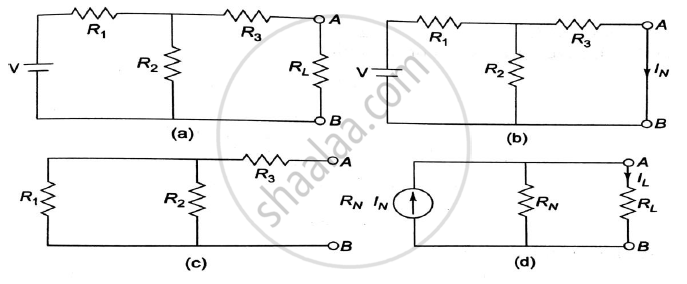# State Norton’S Theorem and Draw the Norton’S Equivalent Circuit. - Basic Electrical and Electronics Engineering

Sum

State Norton’s theorem and draw the Norton’s equivalent circuit.

#### Solution

It states that ‘Any two terminals of a network can be replaced by an equivalent current source and an equivalent parallel resistance.’ The constant current is equal to the current which would flow in a short circuit placed across the terminals. The parallel resistance is the resistance of the network when viewed from these open-circuited terminals after all voltage and current have been removed and replaced by internal resistance.Diagram (d) from above shows the Norton’s equivalent circuit.

Concept: Norton’S Theorem
Is there an error in this question or solution?

Share